# CVX Objective function

How can I write the following CVX objcetive function in a similar way i write in GAMS.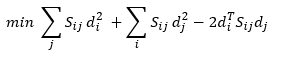Are d_i and d_j scalars, or vectors? The use of the transpose d_i^T suggests a vector, but the use of d_i^2 and d_j^2 suggest scalars. I’m guessing the former, in which case isn’t this just \sum_{i,j} S_{ij} \|d_i-d_j\|_2^2?

My original objective function is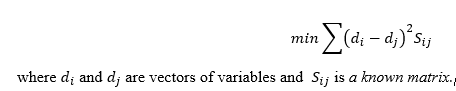Am not sure if this formulation represents the same thing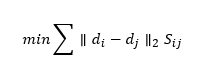The expression (d_i-d_j)^2 is ill-posed if d_i,d_j are vectors. What does that even mean? I think you mean \|d_i-d_j\|_2^2, (and no, not \|d_i-d_j\|_2.)

Yeah that’s what I meant.

So how do i write this in cvx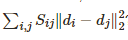Also, I was formulating the problem with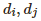,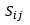scalar values.

Suppose you have a matrix D whose columns represent the vectors d_i, and a matrix S whose values correspond to S_{ij}. Here’s one way to do it:

obj = 0
for i = 1:size(S,1)
for j = 1:size(S,2)
obj = obj + sum_square(D(:i)-D(:,j)) * S(i,j)
end
end
minimize(obj)


This is certainly the easiest way, but depending on the number of vectors, it might be a little slow. There are some vectorization tricks to play here once you confirm this is the right general idea.